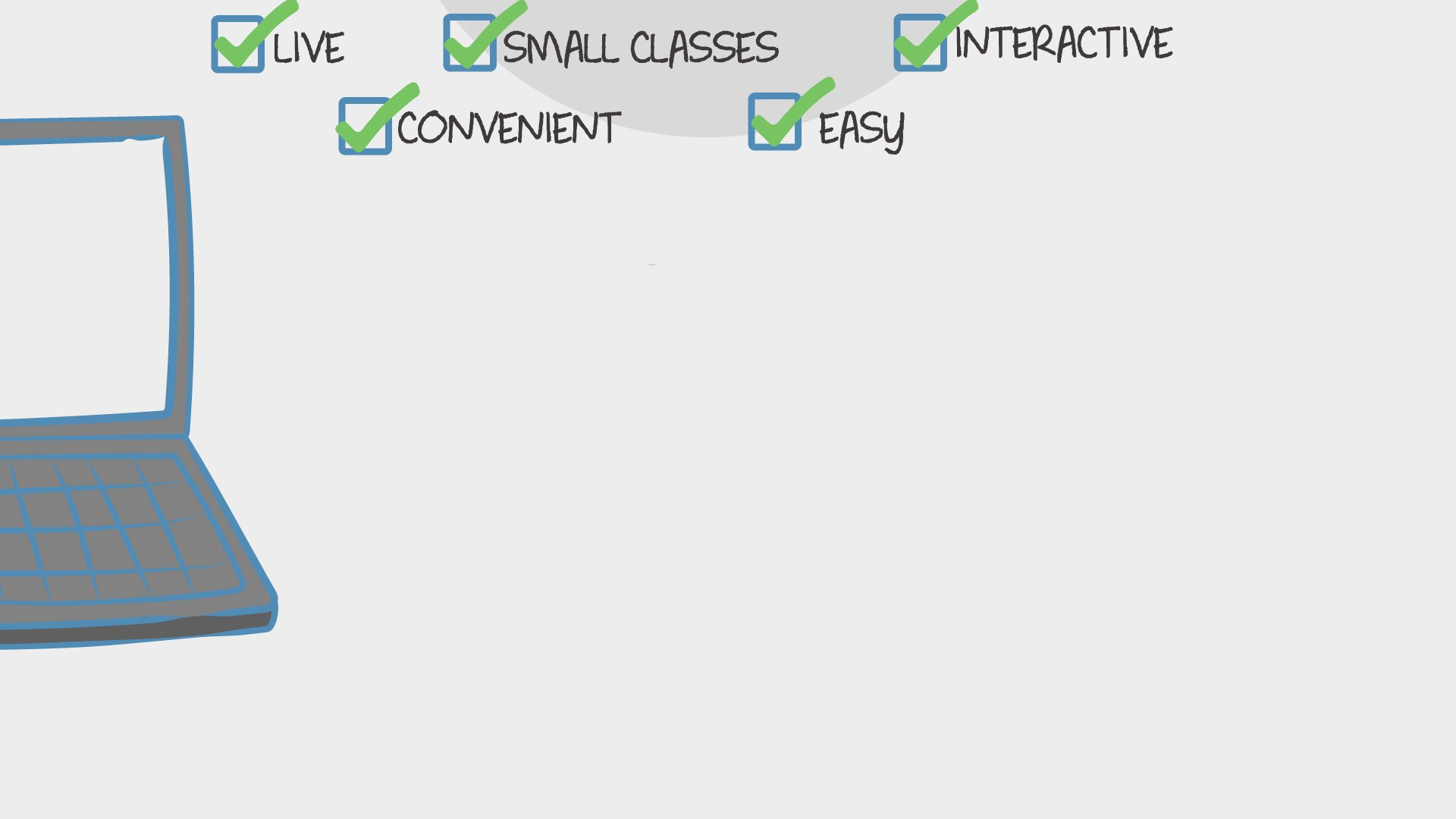Find Classes
Teach

Math

# 4th Grade Math (2nd Quarter)

In this 8 week long class, we will meet all the common core math standards for the 2nd quarter of 4th grade.543 total reviews for this teacher
New class
There are no upcoming classes.40 minutes
per class
Twice per week
over 8 weeks
8-12
year olds
2-6
learners per class
per learner - per class

### How does a “Multi-Day” course work?

Meets multiple times at scheduled times
Live video chats, recorded and monitored for safety and quality
Discussions via classroom forum and private messages with the teacher
Great for engaging projects and interacting with diverse classmates from other states and countries### How Outschool Works### There are no open spots for this class.

You can request another time or scroll down to find more classes like this.

## Description

### Class Experience

```4.OA.1 Interpret a multiplication equation as a comparison, e.g., interpret 35 = 5 × 7 as a statement
that 35 is 5 times as many as 7 and 7 times as many as 5. Represent verbal statements of
multiplicative comparisons as multiplication equations.

4.OA.2 Multiply or divide to solve word problems involving multiplicative comparison, e.g., by using
drawings and equations with a symbol for the unknown number to represent the problem,
distinguishing multiplicative comparison from additive comparison. (See CCLS Glossary, Table
2.)

4.OA.3 Solve multistep word problems posed with whole numbers and having whole-number
answers using the four operations, including problems in which remainders must be
interpreted. Represent these problems using equations with a letter standing for the
unknown quantity. Assess the reasonableness of answers using mental computation and
estimation strategies including rounding.

4.OA.4 Find all factor pairs for a whole number in the range 1–100. Recognize that a whole number is
a multiple of each of its factors. Determine whether a given whole number in the range
1–100 is a multiple of a given one-digit number. Determine whether a given whole number in
the range 1–100 is prime or composite.

4.NBT.5 Multiply a whole number of up to four digits by a one-digit whole number, and multiply two
two-digit numbers, using strategies based on place value and the properties of operations.
Illustrate and explain the calculation by using equations, rectangular arrays, and/or area
models.

4.NBT.6 Find whole-number quotients and remainders with up to four-digit dividends and one-digit
divisors, using strategies based on place value, the properties of operations, and/or the
relationship between multiplication and division. Illustrate and explain the calculation by
using equations, rectangular arrays, and/or area models.

4.MD.3 Apply the area and perimeter formulas for rectangles in real world and mathematical
problems. For example, find the width of a rectangular room given the area of the flooring
and the length, by viewing the area formula as a multiplication equation with an unknown
factor.```
`Students will need paper and pencil or white board and marker for each class.`
`Students will be assessed by observation. We will also have 2 assessments throughout the quarter to see what skills the students have mastered and which skills we should practice more.`
`1 hour 20 minutes per week in class, and maybe some time outside of class.`
`This class will move at the pace of the students. If all students understand a skill we will move on. If students need more practice with a certain skill we will continue to practice before moving on. My goal is for students to master each skill before we move on. If we need to we will move on and then circle back to the skill later to relearn the missing skill.`
`I use common core/ Eureka Math standards to make this curriculum.`

## Teacher

`Hi, my name is Madison. I have a Bachelor's of Science Degree in Elementary Education with an emphasis in Social Science and Math. I have a 5 year old son and a two year old daughter. We love to play outside and create art with the things we find... `